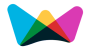#Rule of Three (3 Rule) Calculator

The result is to B what X is to A.## Rule of Three Formulas

The rule of three to calculate the corresponding values of many sizes proportionally.It is also known as Direct Proportion

Direct Proportion: Direct proportion means that any increase in the known values increases the unknown value at the same rate

This Direct Proportion or Rule of three calculation is capable of applying the following formulas:

y = (b × x) / a

Where,

a = Value of a

b = Value of b

x = New Value of a

y = New Value of b

Example :

When you do the shopping, a packet of 500 gram of sugar is worth 2\$. Should this rate there, how much cost a packet of 5 kg?

###More Calculators ►

Online math number calculation, formulas